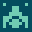# recognizers

#### A collection of recognizers

```\ recognize a time information in the format
\ hh:mm:ss (two : between numbers)
\ returns either rectype-null (if unsuccessful) or
\ a double number representing the seconds of the
\ time stamp. A double number is used to make
\ 16bit systems able to deal with the number of
\ seconds a day has (86400).

\ append it to the recognizer stack
\ and than enter 02:00:00 to get 7200. (double
\ cell number) which is the number of seconds
\ 2 hours have.

S" Recognizer.4th" INCLUDED

\ some factors.
\ is it a : ?
over >r 1 /string r> c@ [char] : = ;

\ extract a number from the current string
0. 2swap >number ;

\ (hours*60+minutes)*60+seconds
: a+60b 2swap #60 1 m*/ d+ ;

: rec-h:m:s ( c-addr u -- d rectype-dnum | rectype-null )
get-number ( -- hh. addr len )
':'? 0= if 2drop 2drop rectype-null exit then

get-number ( -- hh. mm. addr+1 len-1 )
2>r a+60b  2r>
':'? 0= if 2drop 2drop rectype-null exit then

get-number \ -- (hh*60+mm). ss. addr len
\ len must now be 0 or it is not a time stamp
if drop 2drop 2drop rectype-null exit then drop
\ add minutes to seconds and finish
a+60b  rectype-dnum
;

\ wishlist:
\ validate the values for minutes and seconds (between 0 and 59)
\ factor the code

\ tests are made outside of the interpreter, thus the
\ need for explicit strings.

VERBOSE ON

decimal

T{ S" 01:00:00" rec-h:m:s -> #3600. RECTYPE-DNUM }T
T{ S" 01:00:0a" rec-h:m:s -> RECTYPE-NULL }T
T{ S" 72:00:09" rec-h:m:s -> #259209. RECTYPE-DNUM }T

```

## by mtrute